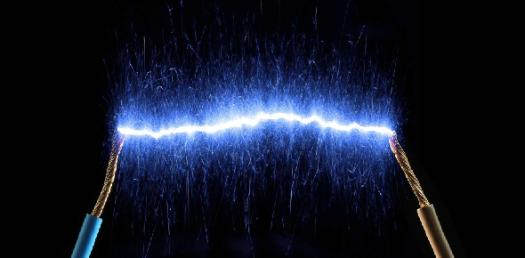# Chapter 7 Electric Current

22 Questions | Total Attempts: 1975SettingsCreate your own QuizChapter 7 electric current

• 1.
The current in a circuit equals the voltage difference divided by the resistance.
• A.

Law of resistance

• B.

Law of compliance

• C.

Ohms Law

• D.

Conductivity Law

• E.

Static electricity

• 2.
A source of voltage difference, such as a battery, a device such as a lightbulb that has resistance and conductors that connects the device to the battery terminals.
• A.

Ohms Law

• B.

Simple circuit

• C.

Flow resistance

• D.

Electric circuit

• E.

Batteries

• 3.
A way to measure the resistance of objects and materials.
• A.

Electric current

• B.

Simple circuit

• C.

Ohms Law

• D.

Voltage difference

• E.

Static electricity

• 4.
Electric current loses energy as it moves through the filament because the filament ____ the flow of ____.
• A.

Electrons, resists

• B.

Protons, inhibits

• C.

Inhibits, electrons

• D.

Resists, electrons

• E.

Contributes, electons

• 5.
How does Ohms Law work?
• A.

An object is connected to a source of filament and the current flowing in the circuit is released.

• B.

An object is connected to a source of voltage difference and the current flowing in the circuit is measured.

• C.

An object is disconnected to a source of voltage difference and the current flowing in the circuit is measured.

• D.

An object is connected to a source of electric energy and the current flowing in the circuit is constricted.

• 6.
Resistance is the tendency for a material to _____ the flow of _____ changing electrical energy into ____ energy and light.
• A.

Oppose, electrons, thermal

• B.

Thermal, protons, oppose

• C.

Oppose, neutrons, thermal

• D.

Thermal, electrons, oppose

• E.

Oppose, light, thermal

• 7.
Six wet cells made up of lead and lead dioxide plates in a sulfuric acid solution.
• A.

Wet cell batteries

• B.

Resistance

• C.

• D.

Terminal batteries

• E.

Dry cell batteries

• 8.
Lead acid batteries provides a voltage difference of ___ volts.
• A.

10

• B.

12

• C.

15

• D.

20

• E.

5

• 9.
Part of the circuit through the bulb is a thin wire called a ______.
• A.

Filament

• B.

Proton

• C.

Battery

• D.

Electron

• E.

Solution

• 10.
As the electrons flow through the filament, they bump into the metal atoms that make up the filament.  In these collisions, some of the electrical energy of the electrons is converted into ______ ______.  This is called _______.
• A.

Resistance, thermal energy

• B.

Energy, thermal resistance

• C.

Cpnductor, resistance

• D.

Thermal energy, resistance

• E.

Wet cells, thermal energy

• 11.
Contains two connected plates made of different metals or metallic compounds in a conducting solution.
• A.

• B.

Dry cell battery

• C.

Wet cell battery

• 12.
What happens to two terminals of a dry-cell battery connected in a circuit involving zinc and several chemicals in the paste.
• A.

Protons are transferred between some of the compounds in this chemical reaction.

• B.

The chemical reaction in each cell provides a voltage difference

• C.

Neutrons are transferred between some of the compounds in this chemical reaction.

• D.

Electrical energy of the electrons is converted into thermal energy

• E.

Electrons are transferred between some of the compounds in this chemical reaction.

• 13.
The net movement of electric charges in a singe direction.
• A.

Voltage difference

• B.

Electric current

• C.

Electric circuits

• D.

Batteries

• 14.
T  or  FThere is no met movement of electrons in one direction.
• A.

True

• B.

False

• 15.
A  ____ ____ is related to the force that causes electric charges to flow.
• A.

Electric currents

• B.

Voltage difference

• C.

Dry cell batteries

• D.

Current flow

• 16.
Voltage difference is measured in _____.
• A.

Amps

• B.

Electrodes

• C.

Volts

• D.

Centimeters

• 17.
A closed path that electric current follows is?
• A.

Electric Circuits

• B.

Resistance

• C.

Batteries

• D.

Voltage Difference

• 18.
A _____ can provide the voltage difference that is needed to keep current flowing in a circuit.
• A.

Current

• B.

Electrode

• C.

Chemical

• D.

Battery

• 19.
T  or F Current flows as long as there is a closed path that connects one battery terminal to the other battery terminal.
• A.

True

• B.

False

• 20.
A cell consists of two electrodes surrounded by a material called an ________.
• A.

Electrode

• B.

Electricity

• C.

Electronic

• D.

Evaluation

• E.

Electrolyte

• 21.
T or FThe electrolyte enables charges to move from one electrode to the other.
• A.

True

• B.

False

• 22.
T  or FWet cell batteries contain electrolytes which contains the least amount of paste chemicals.
• A.

True

• B.

False

Related TopicsBack to top
×

Wait!
Here's an interesting quiz for you.